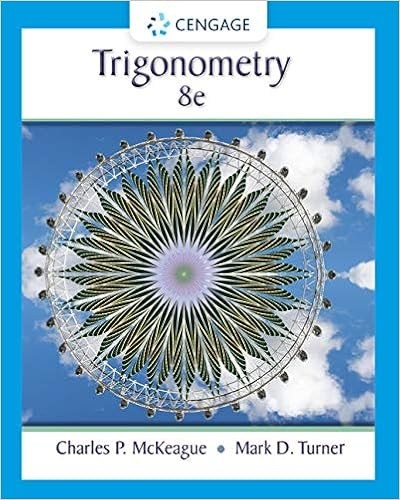# For the mechanism shown at right the slotted bar

• Notes
• KidHackerApe10335
• 32

This preview shows page 25 - 32 out of 32 pages.

##### We have textbook solutions for you!
The document you are viewing contains questions related to this textbook.The document you are viewing contains questions related to this textbook.
Chapter 8 / Exercise A
Trigonometry
McKeague/TurnerExpert Verified
For the mechanism shown at right, the slotted bar rotates with constant angular velocity ϖ= 10 rad/s and the radial position of point A is controlled by the shape of the cam. The path is described by the following equation:r= 1 + 0.5 cos 2θfeet What is the acceleration of point A when θ= 30o?
##### We have textbook solutions for you!
The document you are viewing contains questions related to this textbook.The document you are viewing contains questions related to this textbook.
Chapter 8 / Exercise A
Trigonometry
McKeague/TurnerExpert VerifiedEquations for Circular Motionθrv=θθvran===22rvθrvat==
Look at problem 2/237 on page 112. If the centrifuge spins at a constant angular velocity, then the linear acceleration of the person inside..is in the direction of motionis towards the centeris zerois only in the direction of gravity (downwards)
QUIZ2.The radial component of acceleration of a particle moving in a circular path is always1.The radial component of velocity of a particle moving in a circular path is always
.
To do for next timeWill do more polar and path problemsHomework due FridayLook at EX6.1-2 example problems on BlackboardSet Up IC6.1 with Given and Find for class.Come with questions!
•••